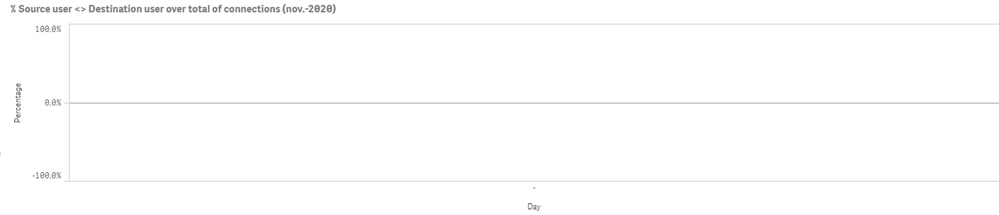# App Development

Discussion board where members can learn more about Qlik Sense App Development and Usage.

Announcements
April 22, 2PM EST: Learn about GeoOperations in Qlik Sense SaaS READ MORE
cancel
Showing results for
Search instead for
Did you mean:Partner

## Not showing current month

Hi all,

I want to show in a line chart the behavior of a KPI during the days of the current month. My expression is as follows:

=1+(

(count({\$<flag_user={"\$(='Sí')"},Month={"\$(=max(Month))"},Year={"\$(=max(Year))"}>}connect_time)
-
count({\$<Month={"\$(=max(Month))"},Year={"\$(=max(Year))"}>}connect_time)

)
/
count({\$<Month={"\$(=max(Month))"},Year={"\$(=max(Year))"}>}connect_time))

It works but only if a select the current month (november) in the filter pane, if not it shows up a  blank.

Without filters:November selected:So I want this last chart to show up without any selections.

Could anyone help me?

Thank you in advance.

Labels (3)

• ### Set Analysis

1 Solution

Accepted SolutionsPartner

It seems that you have value for December for "Month" field (may be due to master calendar).

Just try changing your expression with something like this (calculating current month through today function)

=1+(

(count({\$<flag_user={"\$(='Sí')"},Month={"\$(=month(Today()))"},Year={"\$(=year(Today()))"}>}connect_time)
-
count({\$<Month={"\$(=month(Today()))"},Year={"\$(=year(Today()))"}>}connect_time)

)
/
count({\$<Month={"\$(=month(Today()))"},Year={"\$(=year(Today()))"}>}connect_time))

Hope it helps

Regards

2 RepliesPartner

It seems that you have value for December for "Month" field (may be due to master calendar).

Just try changing your expression with something like this (calculating current month through today function)

=1+(

(count({\$<flag_user={"\$(='Sí')"},Month={"\$(=month(Today()))"},Year={"\$(=year(Today()))"}>}connect_time)
-
count({\$<Month={"\$(=month(Today()))"},Year={"\$(=year(Today()))"}>}connect_time)

)
/
count({\$<Month={"\$(=month(Today()))"},Year={"\$(=year(Today()))"}>}connect_time))

Hope it helps

RegardsPartner
Author

Great Forte! It works!

Good workaround.

Thank you for your help. Regards.